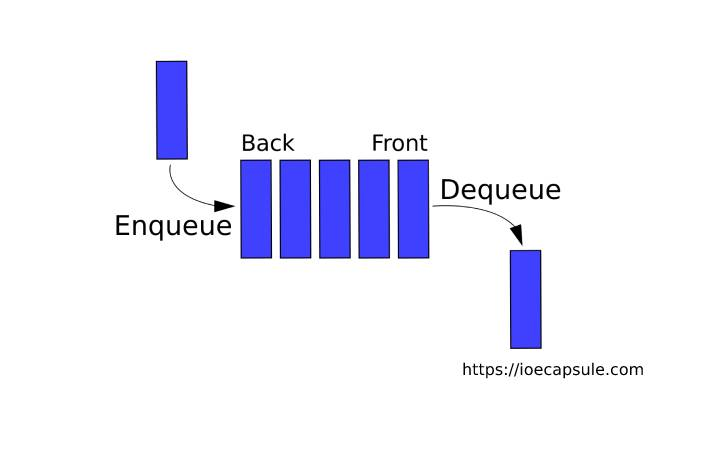### Queue: Template Implementation Of Dynamic Linear Queue Using C++Data Structures

A Queue is a linear, ordered collection of items or data structure in which the operations are performed in First In First Out fashion . Meaning, it is always the first item to be put into the queue, and first item is to be removed first .

If you want to know about algorithms and basics about linear queue, check this out first :

### Queue: Linear Queue Implementation

#### Template Implementation Of Dynamic Linear Queue Using C++

Here, we are using array to add and remove items dynamically. The program is as follows :

``````
#include<iostream>
using namespace std;

template<typename T> class Queue{
private:
T* arr;
int fIndex, rIndex;
public:
Queue(){
fIndex = 0;
rIndex = -1;
arr = NULL;
}

bool empty(){
return rIndex < fIndex;
}

void enqueue(T item){
if(empty()){
this->arr = new T[++this->rIndex + 1];
*(this->arr + this->rIndex) = item;
}else{
//making old copy
T* temp = new T[this->rIndex + 1];
for(int i = 0; i <= this->rIndex; i++){
*(temp + i) = *(this->arr + i);
}

//re-creating array
this->arr = new T[++this->rIndex + 1];
for(int i = 0; i <= this->rIndex-1; i++){
*(this->arr + i) = *(temp + i);
}
*(this->arr + this->rIndex) = item;
}
}

void dequeue(){
if(empty()){
cout<<"queue is empty"<<endl;
return;
}
cout<<*(this->arr+this->fIndex++)<<" dequeued"<<endl;
}

T front(){
if(empty()){
return NULL;
}
return *(arr+fIndex);
}

~Queue(){
delete arr;
}
};

int main(){
bool quit = false;
Queue<int> queue;
int temp;
do{
cout<<"===================================="<<endl;
cout<<"select option :"<<endl;
cout<<"1 for enqueue"<<endl;
cout<<"2 for dequeue"<<endl;
cout<<"3 for front item"<<endl;
cout<<"4 for exit"<<endl;
int ch;
cin>>ch;
cout<<"===================================="<<endl;
switch (ch)
{
case 1:
cout<<"enter item to enqueue:"<<endl;
cin>>temp;
queue.enqueue(temp);
break;
case 2:
queue.dequeue();
break;
case 3:
if(queue.empty()){
cout<<"queue is empty"<<endl;
}else{
cout<<"front: "<<queue.front()<<endl;
}
break;
case 4:
quit = true;
break;
default:
cout<<"invalid selection"<<endl;
break;
}
}while(!quit);
return 0;
}``````